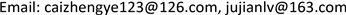1广东技术师范学院电子与信息学院，广东 广州

2广州数字内容处理及其安全性技术重点实验室，广东 广州1. 引言

2. 相关知识2.1. 多假设预测方法

w ^ i = arg min w i ‖ y i c s − Φ H i w i ‖ 2 + λ ‖ Γ w i ‖ 2 (1)

Γ = [ ‖ y i c s − Φ h 1 ‖ 2 0 ⋱ 0 ‖ y i c s − Φ h k ‖ 2 ] (2)

w ^ i = [ ( Φ H i ) T ( Φ H i ) + λ Γ T Γ ] − 1 ( Φ H i ) T y i c s (3)

w ^ i o p t = [ ( Φ H i o p t ) T ( Φ H i o p t ) + λ Γ T Γ ] − 1 ( Φ H i o p t ) T y i c s (4)

2.2. 最佳线性估计方法

L = R x x Φ B T ( Φ B R x x Φ B T ) − 1 (5)

x ^ = L y (6)

R x x = E [ x x T ] = [ E ( x 1 x 1 ) E ( x 1 x 2 ) ⋯ E ( x 1 x B 2 ) E ( x 2 x 1 ) E ( x 2 x 2 ) ⋯ E ( x 2 x B 2 ) ⋮ ⋮ ⋱ ⋮ E ( x B 2 x 1 ) E ( x B 2 x 2 ) ⋯ E ( x B 2 x B 2 ) ] (7)

R x x 矩阵的元素可根据文献  由下式进行估计：

R x x ( p , q ) = E [ x p x q ] = ρ μ (8)

μ = ( n 1 − n 2 ) 2 + ( m 1 − m 2 ) 2 (9)

3. 最佳线性估计与多假设预测相结合的OLE-MH重构算法

Reconstructed PSNR and time for smooth blocks using different algorithm

0.10.20.30.40.5

BCS-SPL-DDWT40.34/1.2543.63/0.8145.60/0.6547.26/0.6748.860.73
BCS-SPL-DCT40.86/0.6043.38/0.7344.95/0.8946.32/1.2347.70/1.56
BCS-TV42.91/52.7344.71/69.1446.17/83.4247.65/98.0849.12/128.95

BCS-SPL-DDWT39.79/1.2144.41/0.8046.24/0.6647.82/0.7249.45/0.70
BCS-SPL-DCT39.83/0.6343.79/0.7645.44/0.9446.94/1.2848.39/1.57
BCS-TV42.38/54.0245.18/71.5746.65/84.3948.04/99.7749.49/131.67

Q i K e y = Φ B K e y × H i (10)

σ = ‖ ( h i current − h ¯ i current ) ‖ 2 N (11)

4. 实验结果与分析4.1. 最佳线性估计方法仿真结果

4.2. 本文提出算法仿真结果

PSNR of Reconstructing non-Key frames using different block-based algorithm

0.10.20.30.40.5
Akiyo
OLE-MH43.6844.3944.6244.7344.84
MH-BCS-SPL38.7541.3342.6043.3343.80
OLE30.3833.9636.9439.6741.96
Silent
OLE-MH35.6636.1136.3036.4236.49
MH-BCS-SPL31.9933.3134.2734.9135.44
OLE27.1829.5831.3833.0534.75
News
OLE-MH37.6638.1038.2538.3538.42
MH-BCS-SPL31.6234.3235.9236.7437.26
OLE24.6627.7930.2732.4134.55

5. 结束语